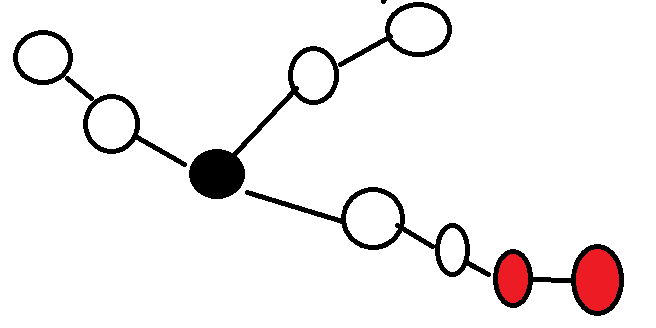# bzoj1095 [ZJOI2007]Hide 捉迷藏

## 1095: [ZJOI2007]Hide 捉迷藏

Time Limit: 40 Sec  Memory Limit: 256 MB
Submit: 5009  Solved: 2072
[Submit][Status][Discuss]

## Description

捉迷藏 Jiajia和Wind是一对恩爱的夫妻，并且他们有很多孩子。某天，Jiajia、Wind和孩子们决定在家里玩

## Input

第一行包含一个整数N，表示房间的个数，房间将被编号为1,2,3…N的整数。接下来N-1行每行两个整数a, b，

## Output

对于每一个操作Game，输出一个非负整数到hide.out，表示最远的两个关灯房间的距离。若只有一个房间是关

8
1 2
2 3
3 4
3 5
3 6
6 7
6 8
7
G
C 1
G
C 2
G
C 1
G

4
3
3
4

## HINT

首先这道题看上去很像数据结构题：多种操作，修改询问什么的都有.

将关着的灯看作黑点，开着的灯看作白点.

如果只有询问操作，是可以dp的. 令f[i][0/1]表示以i为根的子树中的黑点到i的最长距离/次长距离. 类似维护最长链一样，先从下往上求一次，再从上往下求一次. 但是这道题有修改操作，每次修改完以后就必须再做一次dp.复杂度太高.dp这个方法行不通. 这种看上去很像数据结构题的题一般dp是行不通的。

还是考虑只有询问操作.路径问题有没有什么好的方法呢？ 树剖？显然不行吧...... 点分治？emm,似乎可行. 用点分治的话就是类似于bzoj3697的方法了，将树“看作”二叉树，维护前缀信息来做.但你听说过带修改操作的点分治吗?

其实是有这种点分治方法的.一般称之为动态点分治,它维护了一个点分树,修改查询只会在点分树的节点上进行. 点分树的深度是logn的，所以修改查询的复杂度是O(logn). 那么什么是点分树呢？

一般的点分治的题目的步骤是：找重心-在重心节点上dfs-找重心-继续dfs.如果某一次dfs的点是i,下一次dfs的点是j,则i,j连一条边.简而言之，就是把点分治中每个重心的父亲设为它上一层的重心,并在重心处记录下它“管辖”的子树的信息.

点分树特别像线段树，如果说线段树每一个点维护了一段区间的信息，那么点分树中的每一个节点就维护了若干个子树的信息.这对于理解点分树特别有用.

建出点分树很容易，点分树上的每个节点维护什么信息呢？我一开始想的是对每一个节点维护一个大根堆，记录这个节点管辖的点到这个点的距离.每次取出最大和次大的组成一个二元组，再插入到一个全局堆里面. 询问的话直接输出这个全局堆的堆顶就好了. 看起来似乎没问题.但有一种情况不行：黑色点是重心，红色点分别是最长和次长的，查询得到的答案会是3+4 = 7. 事实上它们之间的距离是1.

维护的信息不足以保证正确性.那就多维护点信息呗.对于每个节点维护两个堆.A堆维护这个重心管辖的所有点到父亲重心的距离.

B堆维护它的所有下一层重心的A堆的堆顶. 再开一个全局堆C,维护每一个B堆的最大值+次大值. 比较像线段树维护信息的方式.每次从子节点提取信息.

查询还是一样的，如果点数≥2输出C堆堆顶就好了. 但是修改就非常恶心了,每一次修改会影响当前修改的点  所在层的重心  到点分树的根  的路径上的  所有点.

如果是白点变成黑点.相当于插入了一个黑点嘛.对每一层的重心进行修改. 如果当前的重心的A堆是空的，也就是点全是白点了，那么直接插入A堆即可,并且更改当前重心的父亲的B堆.否则看插入的点是不是A堆中最大的.如果是的话，就要把之前那个A堆中最大的从B堆中删除，维护C堆，然后插入当前值. 否则直接插入就好了.

如果是从黑点变成白点，相当于删除一个点，那么看删除的这个点是不是A堆中权值最大的，如果是，那么就要从B堆中删除它，并维护C堆，否则直接删就好了.

维护B,C堆信息也要讨论.

如果向B堆中插入一个点. B堆中点的数量小于2的话，直接插入B，如果插入了以后点的数量等于2，则将这两个点组成一个二元组插入到C. 如果一开始数量大于2，C堆删掉B堆的最大值+次大值，B堆插入这个值，C堆插入B堆的最大值+次大值.

如果从B堆中删除一个点. 如果B堆中点的数量小于等于2，直接删，如果删了后数量等于1，则从C堆中删掉B堆中剩下的数和已经删掉的这个数（组成不了二元组了）.如果数量大于2，那么先从C堆中删掉B堆的最大值+次大值，然后从B堆中删掉这个数，再向C堆中加入B堆的最大值+次大值.

考虑的过程就是：修改A堆 --> 对B堆造成影响 --> 对C堆造成影响.  另外要注意：C堆中维护的每一个B堆的点数都要≥2.

还有一个问题：优先队列要怎么删数啊？ 一个非常巧妙的做法：再维护一个大根堆！ 如果要删掉x,就将x丢进删除堆中.每次pop或者top操作时，将原堆和删除堆中相同数弹掉（从堆顶开始弹，直到两个堆顶元素不同或者删除堆为空）.

剩下的就是一些细节问题了，总之，这是一道非常变态的题！

#include <cstdio>
#include <queue>
#include <cstring>
#include <iostream>
#include <algorithm>

using namespace std;

const int maxn = 200010;
int n,Q,fa[maxn],dep[maxn],f[maxn],sum,num,sizee[maxn],vis[maxn];

struct node
{
priority_queue <int> p,q;
void push(int x)
{
p.push(x);
}
void erase(int x)
{
q.push(x);
}
void update()
{
while (q.size() && q.top() == p.top())
{
p.pop();
q.pop();
}
}
int top()
{
update();
return p.top();
}
int sec()  //求第二大
{
update();
int x = p.top();
update();
p.pop();
update();
int y = p.top();
update();
p.push(x);
return y;
}
int size()  //容易写错
{
return p.size() - q.size();
}
} A[maxn],B[maxn],C;

{
to[tot] = y;
}

void dfs(int u,int faa)
{
fa[u] = faa;
dep[u] = dep[faa] + 1;
for (int i = head[u]; i; i = nextt[i])
{
int v = to[i];
if (v == faa)
continue;
dfs(v,u);
}
}

void findroot(int u,int faa)
{
sizee[u] = 1;
f[u] = 0;
for (int i = head[u]; i; i = nextt[i])
{
int v = to[i];
if (v == faa || vis[v])
continue;
findroot(v,u);
sizee[u] += sizee[v];
f[u] = max(f[u],sizee[v]);
}
f[u] = max(f[u],sum - sizee[u]);
if (f[u] < f[root])
root = u;
}

int lca(int x,int y)
{
if (dep[x] < dep[y])
swap(x,y);
for (int i = 19; i >= 0; i--)
if (dep[fa[x][i]] >= dep[y])
x = fa[x][i];
if (x == y)
return x;
for (int i = 19; i >= 0; i--)
if (fa[x][i] != fa[y][i])
{
x = fa[x][i];
y = fa[y][i];
}
return fa[x];
}

int dist(int x,int y)
{
return dep[x] + dep[y] - 2 * dep[lca(x,y)];
}

void build(int u)
{
vis[u] = 1;
for (int i = head[u]; i; i = nextt[i])
{
int v = to[i];
if (vis[v])
continue;
f = sum = sizee[v];
findroot(v,root = 0);
fa2[root] = u;
build(root);
}
}

void init()
{
for (int i = 1; i <= n; i++)
{
int now = i;
while (fa2[now])
{
A[now].push(dist(fa2[now],i));
now = fa2[now];
}
}
for (int i = 1; i <= n; i++)
if (fa2[i])
B[fa2[i]].push(A[i].top());
for (int i = 1; i <= n; i++)
if (B[i].size() >= 2)
C.push(B[i].top() + B[i].sec());
}

void update1(int x)
{
int faa = fa2[x];
if (B[faa].size() < 2)
{
B[faa].push(A[x].top());
if (B[faa].size() == 2)
C.push(B[faa].top() + B[faa].sec());
}
else
{
int temp = B[faa].top() + B[faa].sec();
C.erase(temp);
B[faa].push(A[x].top());
C.push(B[faa].top() + B[faa].sec());
}
}

void update2(int x,int y)
{
int faa = fa2[x];
if (B[faa].size() <= 2)
{
B[faa].erase(y);
if (B[faa].size() == 1)
C.erase(B[faa].top() + y);
}
else
{
C.erase(B[faa].top() + B[faa].sec());
B[faa].erase(y);
C.push(B[faa].top() + B[faa].sec());
}
}

void update(int x)
{
if (col[x])
{
int now = x;
while (fa2[now])
{
if (A[now].size() == 0)
{
A[now].push(dist(x,fa2[now]));
update1(now);
}
else
{
int temp = A[now].top();
A[now].push(dist(x,fa2[now]));
if (A[now].top() != temp)
{
update2(now,temp);
update1(now);
}
}
now = fa2[now];
}
}
else
{
int now = x;
while (fa2[now])
{
int temp = dist(x,fa2[now]);
if (temp == A[now].top())
{
A[now].erase(temp);
update2(now,temp);
if (A[now].size())
update1(now);
}
else
A[now].erase(temp);
now = fa2[now];
}
}
col[x] ^= 1;
}

int main()
{
scanf("%d",&n);
for (int i = 1; i < n; i++)
{
int x,y;
scanf("%d%d",&x,&y);
}
dfs(1,0);  //为了求LCA和两点距离
for (int j = 1; j <= 19; j++)
for (int i = 1; i <= n; i++)
fa[i][j] = fa[fa[i][j - 1]][j - 1];
f = sum = num = n;
findroot(1,0);  //找根
build(root);  //建点分树
init();  //初始化所有黑点的信息
scanf("%d",&Q);
while (Q--)
{
char ch;
scanf("%s",ch);
if (ch == 'G')
{
if (num < 2)
printf("%d\n",num - 1);
else
printf("%d\n",C.top());
}
else
{
int x;
scanf("%d",&x);
update(x);
if (col[x])
num--;
else
num++;
}
}

return 0;
}

posted @ 2018-03-07 16:10  zbtrs  阅读(74)  评论(0编辑  收藏